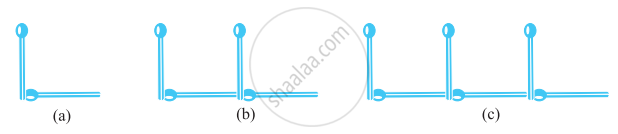Tamil Nadu Board of Secondary EducationSSLC (English Medium) Class 10th

# Introduction to Algebra

## Definition

The branch of mathematics in which we studied numbers is arithmetic.

The branch of mathematics in which we studied shapes is geometry.

The study to use the letters and symbols in mathematics is called Algebra.

## Notes

### Introduction to Algebra:

• There are so many branches of mathematics -
The branch of mathematics in which we studied numbers is arithmetic.
The branch of mathematics in which we studied shapes is geometry.
The study to use the letters and symbols in mathematics is called Algebra.

• The word ‘algebra’ is derived from the title of the book, ‘Aljebar w’al almugabalah’.

• A branch of mathematics in which symbols, usually letters of the alphabet, represent numbers or members of a specified set and are used to represent quantities and to express general relationships that hold for all members of the set.

#### The main feature of algebra: (use of letters)

• The use of letters will allow us to write rules and formulas in a general way.

• By using letters, we can talk about any number and not just a particular number.

• Secondly, letters may stand for unknown quantities. By learning methods of determining unknowns, we develop powerful tools for solving puzzles and many problems from daily life.

• Thirdly, since letters stand for numbers, operations can be performed on them as on numbers. This leads to the study of algebraic expressions and their properties.

### Matchstick Patterns:

The number of matchsticks required to make a pattern of LsNo. of matchsticks used to make 1st L = 2

No. of matchsticks used to make 2nd L = 4

No. of matchsticks used to make 3rd L = 6

So, the pattern that we observe here is 2 × the number of Ls.

If you would like to contribute notes or other learning material, please submit them using the button below.

### Shaalaa.com

Introduction To Algebra [00:12:52]
S
0%